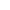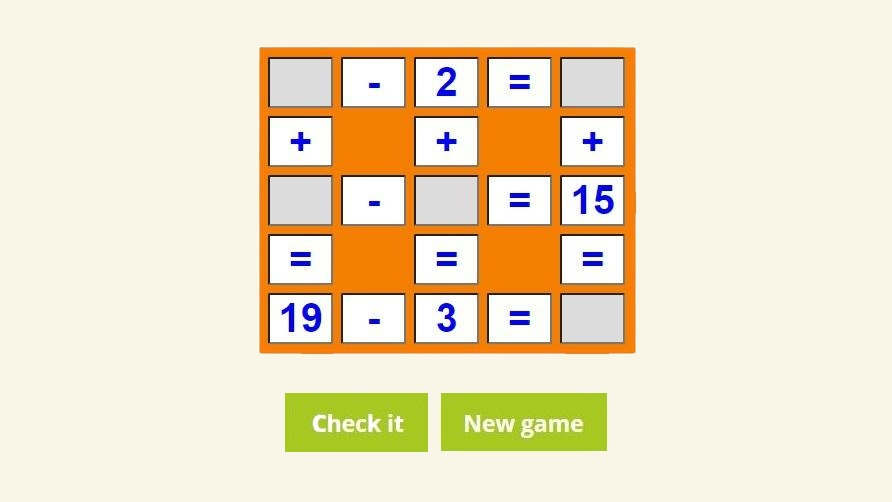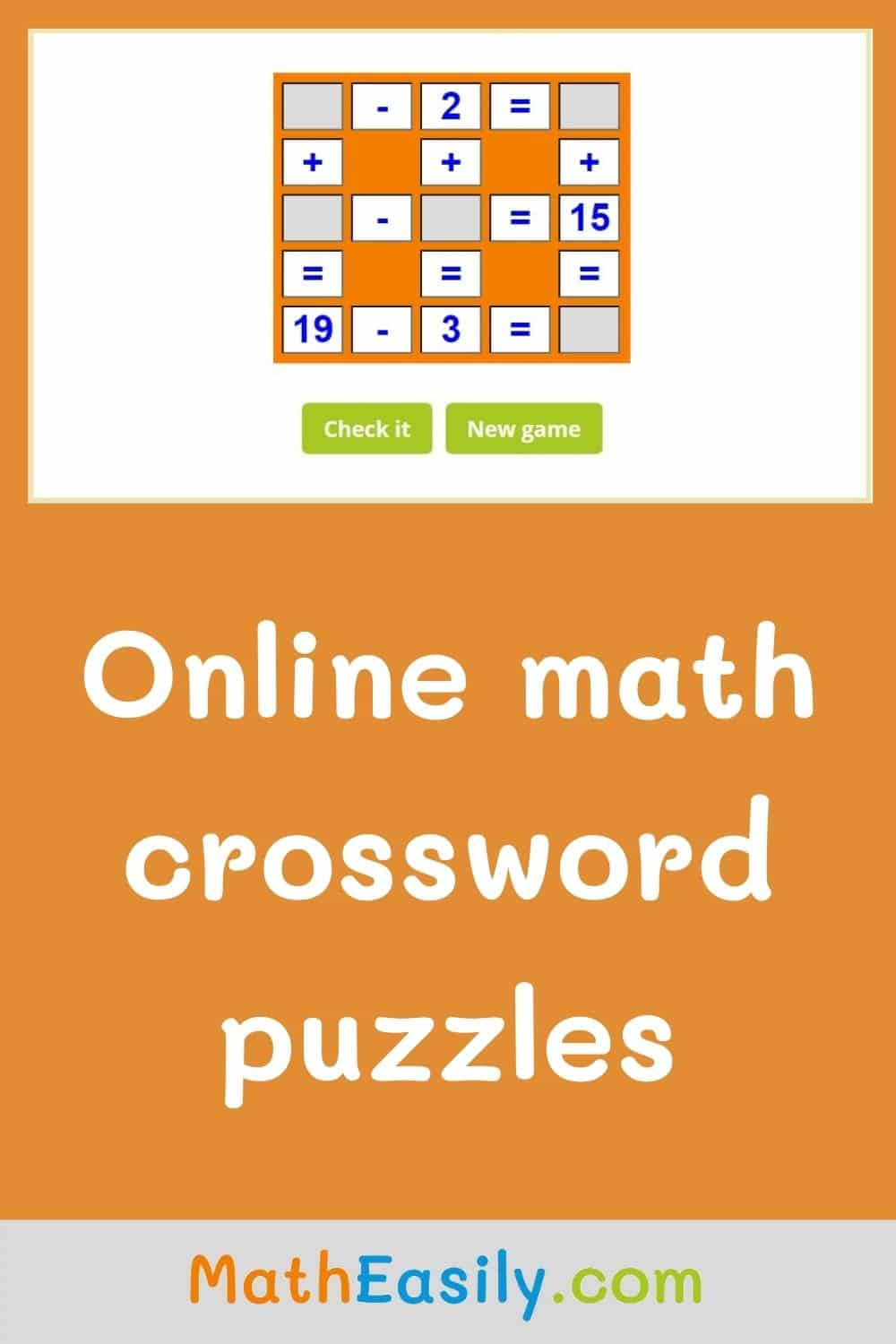﻿ Math crossword puzzles online games + worksheets

Page No. 1045

# Math crossword puzzles

Math crosswords. Free online math crossword puzzles online games. Cross math game online. Free crossword puzzles for math. Free printable number crossword puzzles online free. Maths crossword puzzle games. Cross math puzzles. mathematics crossword puzzle PDF. number crossword online. online crossword puzzle maker. addition and subtraction crossword puzzles. number crossword puzzle maker. addition math crossword puzzle maker. mathematical crosswords. how to solve math crossword puzzles. Free math crossword games. interactive crossword puzzles for kids. cross math online. crossword maths puzzles. number puzzles online. crossmath printable. math crossword puzzles printable.

New gameFill in the blanks so that each equation is correct in the math crossword puzzle. You can choose the maximum value: 20, 30, 50 or 100.

## How to solve math crossword puzzles online?First, look for an operation that you can finish. With the number found you can solve another operation. Continue to do this until all exercises are solved and the number crossword puzzle is completed.

In math crossword puzzles, each equation should be solved from left to right and from top to bottom. If you are not sure how to solve crossword math puzzles correctly, press "Check it" for the first game and you will see the answer key.

### Cross math ONLINE

Fill in all missing numbers so the operations are correct. Play online math crossword puzzles with answers, check results, find mistakes and play again. Do you want to try more difficult whole number crossword puzzles? Then play these addition and subtraction within 100 games:

Look inside

## Free printable math crossword puzzles in PDF

You can generate new math crosswords (as many you want, in order every student in the classroom has its own crossword): just click the green button or you can download ready made free printable math crossword puzzles with answers in PDF below. These worksheets have two pages, the first one is the math crossword puzzle and the other one contains also the answers.

## Why to play number crossword puzzles online?

Math crossword puzzle game is a nice way how to practice addition to 20 and subtraction up to 20 at the same time.

Press "Check it" to find out if the number crossword puzzle is filled in correctly. The correct results will be green, wrong answers will be red. Press the button "New game" and the new online math crossword puzzle will be generated.### Math crossword puzzles maker

I developed this math crossword puzzle maker in spring 2020 when we were hit by Covid 19 epidemic and my kids stayed at home for homeschooling.

My younger son liked math crosswords puzzles very much but but there were only a few worksheets available on the internet. Another problem was, that we didn´t have a printer at home.

I told myself: "It would be nice to have something like unlimited live worksheets, which you can solve online and you will see the right answer immediately."

In that time I didn´t have any knowledge of javascript but I learned what was necessary to develop free math crossword puzzles which can be solved online. Enjoy these free math crosswords as we do!

## Cross math puzzle ONLINE

You can set up your own difficulty level in this crossmath puzzle. From addition and subtraction to 20 to more advanced 2 digit addition and 2 digit subtraction.

When students are familiar with math operations to 100, they can practice these skills by playing magic square puzzles.

## Online math crossword puzzles for kids

To learn math online is fun. These math crosswords these puzzles are the perfect preparation for solving equations and more complex math problems: for example these math matchstick puzzles.

Thousands of new free math crossword puzzles for kids can be generated in the online math crossword puzzles maker. You don´t need to print any worksheet and you see immediately, where you made mistakes.

### Crossword maths puzzles

Addition and subtraction crossword puzzles are super helpful for kids, because they learn math easily when they control their own learning. Read more about it in Psychology Today.

## Cross math ONLINE

Yu can play this cross math game online and save our trees. You need only internet connection to solve this cross math game.

### Cross math game online

Our cross math puzzles are a type of educational activity that combines the principles of traditional crossword puzzles with mathematical concepts.

Instead of using words, these crossword maths puzzles use mathematical equations, operations, and numerical answers to fill in the grid.

Just like traditional crosswords, the goal is to fill in the maths crosswords with the correct numbers that satisfy both the across and down clues.

Category: